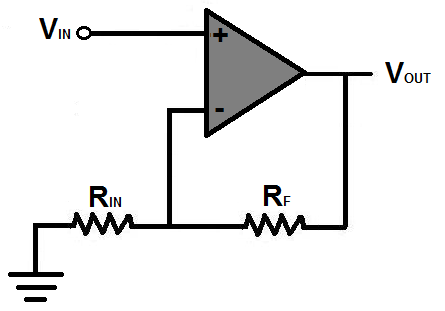# Arduino due ADC time setup

Good morning people!

I use an arduino due to read the voltage values. The read values bounce too much, so the solution has arisen that the integration time of the ADC should be increased. See if it helps. However, I do not find how to solve this.

I welcome other possible solutions. : D
It is very difficult to incorporate filtering into the circuit, so it would be better to look for a solution in the program first.

There are a few ways to do this. What do you mean by the values 'bounce too much'. Do you mean there is a lot of noise? For example, if you try to measure 2.5 volts, does it bounce between 2.4 to 2.6 V, or between 2 to 3 V?

As an initial guess, one technique you can use is averaging. For example, you can copy this function to your code:

``````float smoothedADCreading(uint16_t numOfMeasurements, float ADCreferenceVoltage, uint8_t analogPin){

float result = 0;

for(uint16_t n = 0; n<numOfMeasurements;n++)
{
}

}
``````

from which, assuming you have a 3.3 volt analogue reference voltage, you could read the voltage at A0 1000 times and take the average voltage using:

``````float sensorVoltage = smoothedADCreading(1000, 3.3, A0);
``````

Past this, you could employ some of the techniques in Nick Gammon's ADC examples:

I use a 12-bit scan. The jumps of the values show a deviation of roughly 5-10 values (the read values) which means a deviation of mV.
The trouble is that you have to set a couple of digital pots based on this value and sometimes one jumps to the other because of the bounce.

Averaging helps a bit, but even so, values bounce.
I'll look at the link you sent and try to see if there is any improvement.

Deviation of single mV's is hard to eliminate, especially with a 12-bit ADC.

How often are you reading values? and what are you reading? Is it a thermocouple or some other sensor?

The reason I ask is that your problem may be a hardware issue, i.e. the physical properties of the sensor/circuit, as opposed to something that you can eliminate through code

The time between readings varies but is usually a couple of 100ms. But there is more.
I measure the output voltage of an operational amplifier which I divide by 2 resistors.

how, like this?